# Average - Quantitative Aptitude (MCQ) questions

Dear Readers, Welcome to Quantitative Aptitude Average questions and answers with explanation. These Average solved examples with shortcuts and tricks will help you learn and practice for your Placement Test and competitive exams like Bank PO, IBPS PO, SBI PO, RRB PO, RBI Assistant, LIC,SSC, MBA - MAT, XAT, CAT, NMAT, UPSC, NET etc.

After practicing these tricky Average multiple choice questions, you will be exam ready to deal with any objective type questions.

1)   In the India-Australia one day match, due to rain, India needed 324 runs in 48 overs to win. In initial 10 overs, the average scoring rate was 6, but in next 10 overs it increased to 8.5. It then declined to 5.5 in next 10 overs and again rose to 7 in next 10 overs. To win the match now what average is needed?
- Published on 08 May 17

a. 8.25
b. 6.75
c. 7.75
d. 7.0
 Answer  Explanation ANSWER: 6.75 Explanation: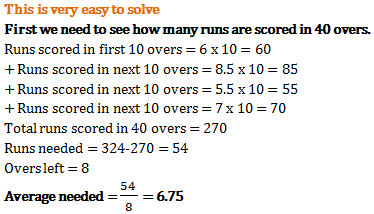2)   Average age of 7 family members is 75 years. But average age of 6 of them is 74 years 6 months. What is the age of the 7th family member?

- Published on 11 Apr 17

a. 75.5
b. 78
c. 68
d. 80
 Answer  Explanation ANSWER: 78 Explanation: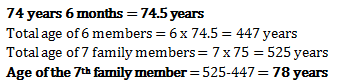3)   Average age of 5 people is 42 years. Another group has 8 people who have average age of 81 years. When both groups are mixed what is average age of all people?
- Published on 11 Apr 17

a. 64 years
b. 66 years
c. 61.5 years
d. 70 years
 Answer  Explanation ANSWER: 66 years Explanation: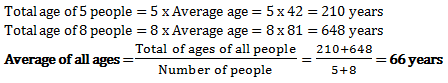4)   Average age of 5 people in a family is 55 years. However it is seen that 3 of the 5 people also have an average age of 55 years. What will be the average age of remaining two people of the family?
- Published on 11 Apr 17

a. 82.5 years
b. 27.5 years
c. 55 years
d. 110 years
 Answer  Explanation ANSWER: 55 years Explanation: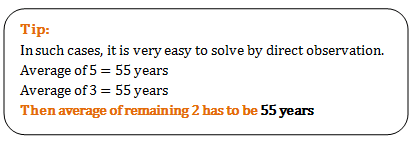5)   Which of the following exactly denotes the average price of all the goods together if, Ramesh buys ‘a’ number of goods of type ‘A’ at price of Rs. ‘E’ each, ‘b’ number of goods of type ‘B’ at price of Rs. ‘F’ each and ‘c’ number of goods of type ‘C’ at price of Rs. ‘G’ each?
- Published on 11 Apr 17

a. (E+F+G) / (a+b+c)
b. (AE+BF+CG) / (a+b+c)
c. (aE+bF+cG) / (a+b+c)
d. (aA+bB+cC) / (a+b+c)
 Answer  Explanation ANSWER: (aE+bF+cG) / (a+b+c) Explanation: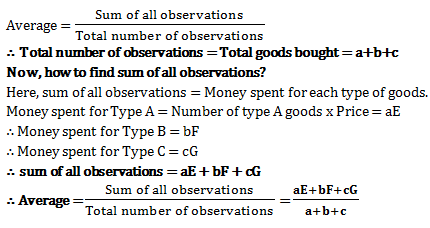6)   The average of fifty numbers is 28. If two numbers, namely 25 and 35 are discarded, the average of the remaining numbers is nearly,
- Published on 07 Jul 17

a. 29.27
b. 27.92
c. 27.29
d. 29.72
 Answer  Explanation ANSWER: 27.92 Explanation: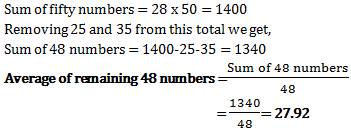7)   The average of three numbers is 77. The first number is twice the second and the second number is twice the third. Find the first number.
- Published on 07 Jul 17

a. 33
b. 66
c. 77
d. 132
 Answer  Explanation ANSWER: 132 Explanation: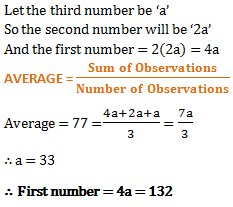8)   Average age of A and B is 30 years, that of B and C is 32 years and the average age of C and A is 34 years. The age of C is
- Published on 07 Jul 17

a. 33 years
b. 34 years
c. 35 years
d. 36 years
 Answer  Explanation ANSWER: 36 years Explanation: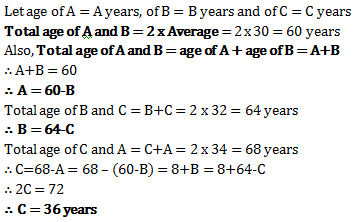9)   3 boxes have some average weight. When one box which weighs 89 kg is replaced by another box, the average weight increases by 5 kg. How much the new box weighs?
- Published on 17 Mar 17

a. 109 kg
b. 94 kg
c. 104 kg
d. 84 kg
 Answer  Explanation ANSWER: 104 kg Explanation: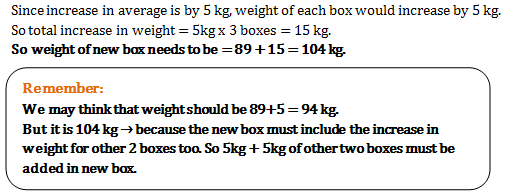10)   Knowing that Vijay’s expenditure for first 3 days is Rs. 100, Rs. 125 and Rs. 85, what is his 4th day expenditure as his 4 days average expenditure Rs. 90?
- Published on 17 Mar 17

a. Rs. 220
b. Rs. 60
c. Rs. 50
d. Rs. 90
 Answer  Explanation ANSWER: Rs. 50 Explanation: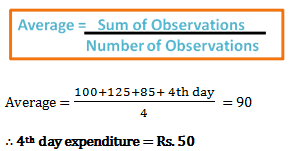1 2 3 4 5